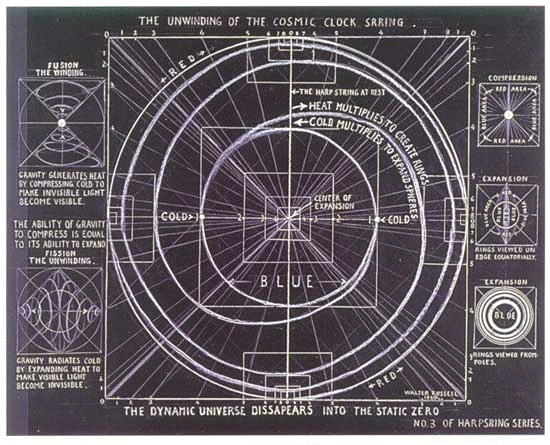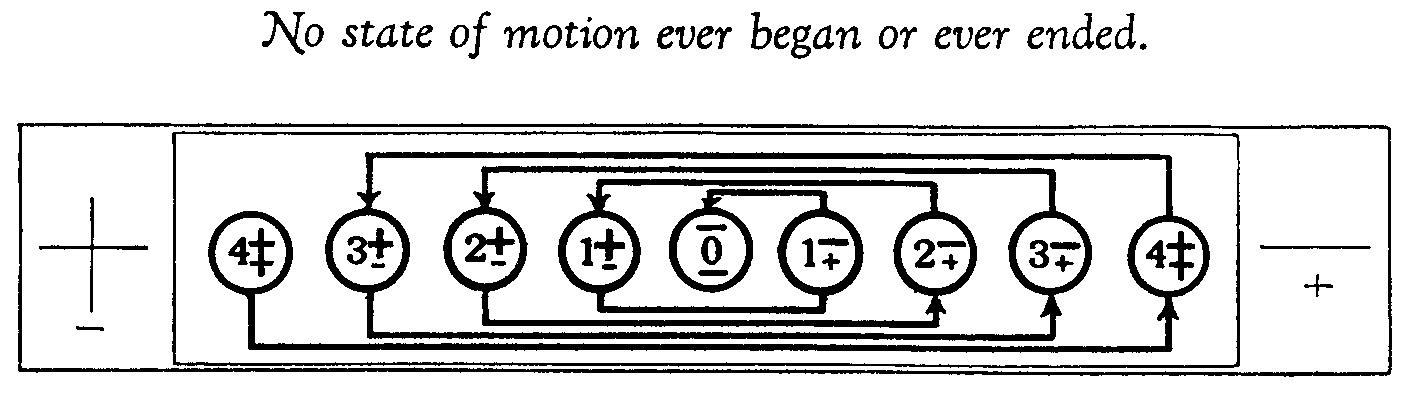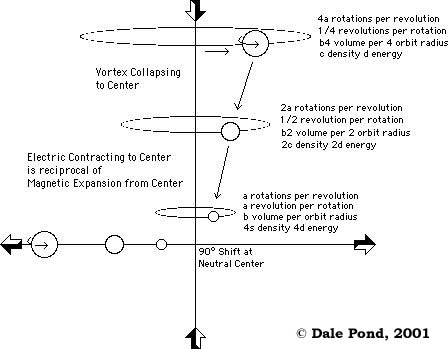# 3.13 - Reciprocals and Proportions of Motions and Substance

"The following fundamental laws governing mathematical relations will briefly convey the idea of the Principles governing universal ratios which is in every effect of motion the direct and inverse square of the distance, area or time dimensions and the cube of volume or potential dimensions. The direct and the inverse are the two way indicators of direction, the latter belonging to gravitational and the former to radiational effects." [Russell, Genero-Radiative Concept, part 6b]

Notice the reference to the Square Law and Inverse Square Law in the above Russell quote. These two laws are reciprocals [see Covariance] of each other and reflect the natural ways force and energy interchange in nature when forming gyroscopic motions or vortices.

"All dimensions simultaneously expand and contract in opposite directions of the same ratio. [Russell, The Universal One, Book 02 - Chapter 14 - Universal Mathematics - Universal Ratios]Figure 3.20 - The Observable Disappears into the Non-observable.
(courtesy University of Science and Philosophy)
(click to enlarge)

"Expansion pressure is in direct ratio to the square of the distance, area, plane, orbit or time unit, and to the cube of the volume.

"Contraction pressure is in inverse ratio to the square of the distance, area, plane, orbit or time unit, and to the cube of the volume." Russell, The Universal One, pg 162

"Every mass in the universe occupies a measurable potential position." Russell, The Universal One, pg 162)

Russell is referring to the Law of Relativity of Force which is indicated by E=mc2 which would be relativity of force when coupled with say Planck's Constant as a universal unit of progression in force/energy. These units of constant force and energy may be added, subtracted, multiplied and divided.

"Every generative effect of motion begins its cycle at its maximum in the region of lowest pressure, loses one half of every dimension of it at the high pressure point of its cycle wave, and exhausts its effect at the place of its beginning.

"Every radiative effect of motion begins its cycle at its minimum in the region of the lowest pressure, gains one-half of every dimension of it at the high pressure point of its cycle wave, and attains its maximum at the place of its ending." [Russell, Genero-Radiative Concept, part 6b]Attraction